# Modeling Mutual Coupling in Large Arrays Using Infinite Array Analysis

This example uses infinite array analysis to model large finite arrays. The infinite array analysis on the unit cell reveals the scan impedance behavior at a particular frequency. This information is used with the knowledge of the isolated element pattern and impedance to calculate the scan element pattern. The large finite array is then modeled using the assumption that every element in the array possesses the same scan element pattern.

This example requires Antenna Toolbox™.

### Define Individual Element

For this example we choose the center of the X-band as our design frequency.

freq = 10e9; vp = physconst('lightspeed'); lambda = vp/freq; ucdx = 0.5*lambda; ucdy = 0.5*lambda; 

Create a thin dipole of length slightly less thanand assign it as the exciter to a infinitely large reflector.

d = dipole; d.Length = 0.495*lambda; d.Width = lambda/160; d.Tilt = 90; d.TiltAxis = [0 1 0]; r = reflector; r.Exciter = d; r.Spacing = lambda/4; r.GroundPlaneLength = inf; r.GroundPlaneWidth = inf; figure; show(r);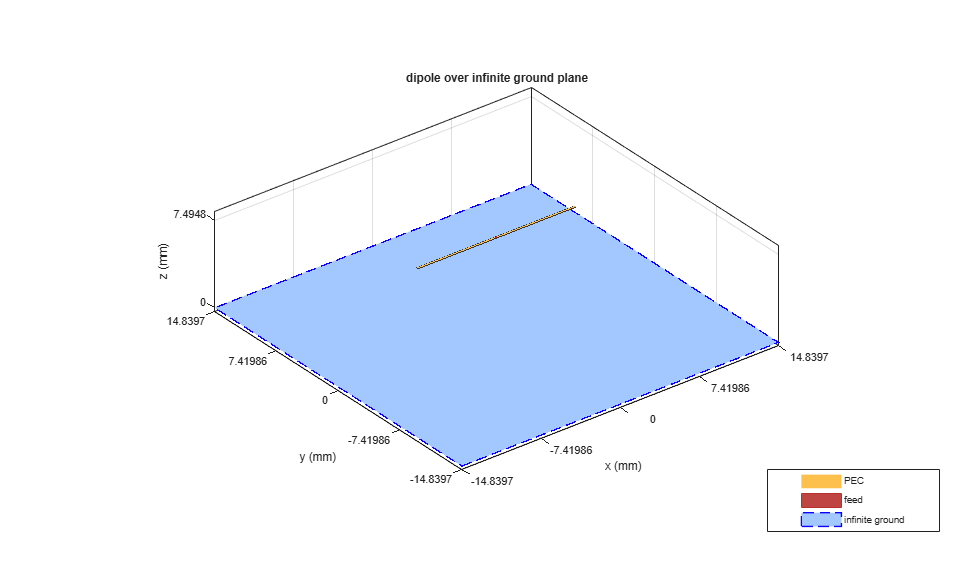Calculate the isolated element pattern and the impedance of the above antenna. These results will be used to calculate the Scan Element Pattern(SEP). This term is also known as Array Element Pattern(AEP) or Embedded Element Pattern(EEP).

%Define az and el vectors az = 0:2:360; el = 90:-2:-90; % Calculate power pattern giso = pattern(r,freq,az,el,'Type','power'); % Calculated impedance Ziso = impedance(r,freq); 

### Calculate Infinite Array Scan Element Pattern

Unit Cell In the infinite array analysis the term unit cell refers to a single element in an infinite array. The unit cell element needs a ground plane. Antennas that don't have a ground plane need to be backed by a reflector. A representative example for each case would be dipole backed by a reflector and a microstrip patch antenna. This example will use the dipole backed by a reflector and analyze the impedance behavior at 10 GHz as a function of scan angle. The unit cell will have axcross-section.

r.GroundPlaneLength = ucdx; r.GroundPlaneWidth = ucdy; infArray = infiniteArray; infArray.Element = r; infArray.ScanAzimuth = 30; infArray.ScanElevation = 45; figure; show(infArray);Scan impedance The scan impedance at a single frequency and single scan angle is shown below.

scanZ = impedance(infArray,freq) 
scanZ = 1.1077e+02 + 3.0010e+01i 

For this example the scan impedance for the full volume of scan is calculated using 50 terms in the double summation for the periodic Greens function to improve convergence behavior.

Scan Element Pattern /Array Element Pattern /Embedded Element Pattern The scan element pattern (SEP) is calculated from the infinite array scan impedance, the isolated element pattern and the isolated element impedance. The expression used is shown here,:load atexInfArrayScanZData scanZ = scanZ.'; Rg = 185; Xg = 0; Zg = Rg + 1i*Xg; gs = nan(numel(el),numel(az)); for i = 1:numel(el) for j = 1:numel(az) gs(i,j) = 4*Rg*real(Ziso).*giso(i,j)./(abs(scanZ(i,j) + Zg)).^2; end end 

### Build Custom Antenna Element

The scan element pattern which represents a power pattern is used to build a custom antenna element.

fieldpattern = sqrt(gs); bandwidth = 500e6; customAntennaInf = helperATXBuildCustomAntenna(... fieldpattern,freq,bandwidth,az,el); figure; pattern(customAntennaInf,freq);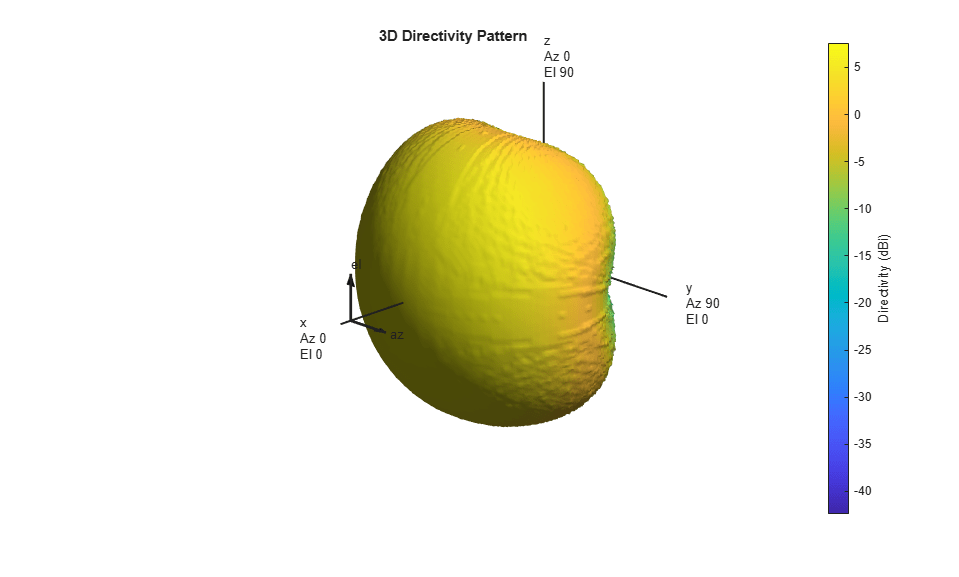### Build 21 X 21 URA

Create a uniform rectangular array (URA), with the custom antenna element, which has the scan element pattern.

N = 441; Nrow = sqrt(N); Ncol = sqrt(N); drow = ucdx; dcol = ucdy; myURA1 = phased.URA; myURA1.Element = customAntennaInf; myURA1.Size = [Nrow Ncol]; myURA1.ElementSpacing = [drow dcol]; 

### Plot Slices in E and H Planes

Calculate the pattern in the elevation plane (specified by azimuth = 0 deg and also called the E-plane) and azimuth plane (specified by elevation = 0 deg and called the H-plane) for the array built using infinite array analysis.

azang_plot = -90:0.5:90; elang_plot = -90:0.5:90; % E-plane Darray1_E = pattern(myURA1,freq,0,elang_plot); Darray1_Enormlz = Darray1_E - max(Darray1_E); % H-plane Darray1_H = pattern(myURA1,freq,azang_plot,0); Darray1_Hnormlz = Darray1_H - max(Darray1_H); % Scan element pattern in both planes DSEP1_E = pattern(customAntennaInf,freq,0,elang_plot); DSEP1_Enormlz = DSEP1_E - max(DSEP1_E); DSEP1_H = pattern(customAntennaInf,freq,azang_plot,0); DSEP1_Hnormlz = DSEP1_H - max(DSEP1_H); 
figure subplot(211) plot(elang_plot,Darray1_Enormlz,elang_plot,DSEP1_Enormlz,'LineWidth',2) grid on axis([min(azang_plot) max(azang_plot) -40 0]); legend('Array Pattern, az = 0 deg','Element Pattern') xlabel('Elevation (deg)') ylabel('Directivity (dB)') title('Normalized Directivity') subplot(212) plot(azang_plot,Darray1_Hnormlz,azang_plot,DSEP1_Hnormlz,'LineWidth',2) grid on axis([min(azang_plot) max(azang_plot) -40 0]); legend('Array Pattern, el = 0 deg','Element Pattern') xlabel('Azimuth (deg)') ylabel('Directivity (dB)')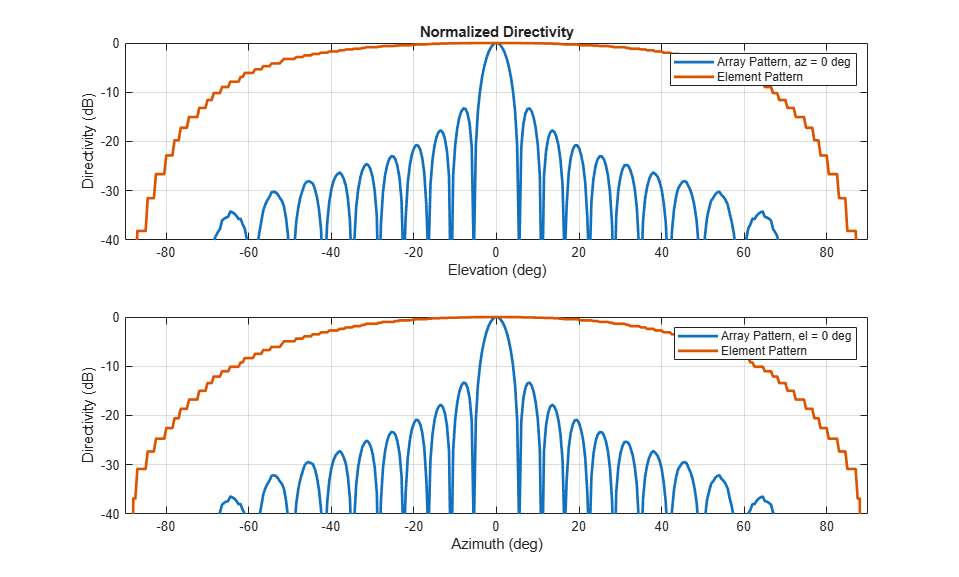### Comparison with a Full Wave Finite Array Analysis

To understand the effect of the finite size of the array, we execute a full wave analysis of a 21 X 21 dipole array backed by an infinite reflector. The full wave array pattern slices in the E and H planes as well as the center element embedded element pattern is also calculated. This data is loaded from a MAT file. This analysis took approximately 630 seconds on a 2.4 GHz machine with 32 GB memory.

Load Full Wave Data and Build Custom Antenna Load the finite array analysis data, and use the embedded element pattern to build a custom antenna element. Note that the pattern from the full-wave analysis needs to be rotated by 90 degrees so that it lines up with the URA model built on the YZ plane.

load atexInfArrayDipoleRefArray elemfieldpatternfinite = sqrt(FiniteArrayPatData.ElemPat); arraypatternfinite = FiniteArrayPatData.ArrayPat; bandwidth = 500e6; customAntennaFinite = helperATXBuildCustomAntenna(... elemfieldpatternfinite,freq,bandwidth,az,el); figure pattern(customAntennaFinite,freq)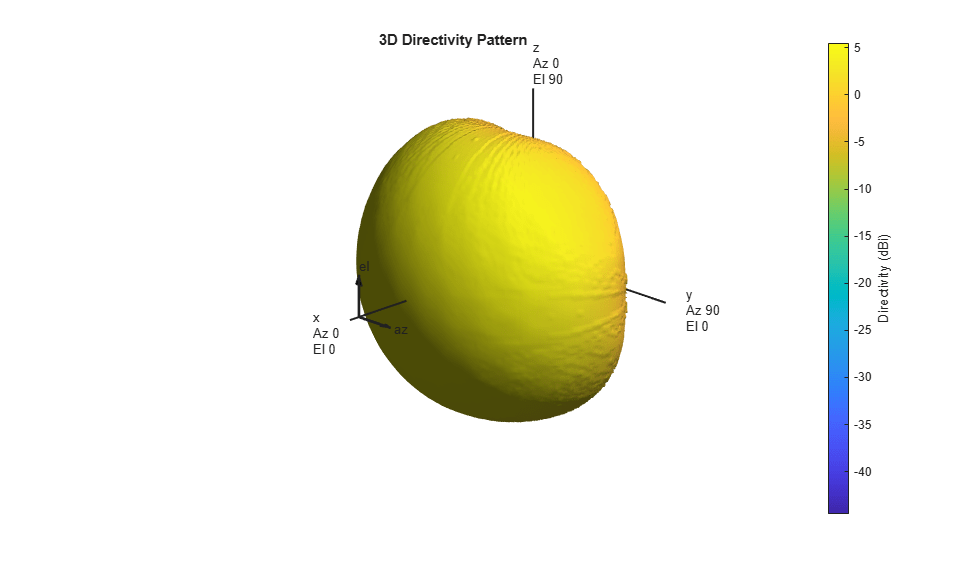Create Uniform Rectangular Array with Embedded Element Pattern As done before create a uniform rectangular array with the custom antenna element.

myURA2 = phased.URA; myURA2.Element = customAntennaFinite; myURA2.Size = [Nrow Ncol]; myURA2.ElementSpacing = [drow dcol]; 

E and H Plane Slice - Array With Embedded Element Pattern Calculate the pattern slices in two orthogonal planes - E and H for the array with the embedded element pattern and the embedded element pattern itself. In addition since the full wave data for the array pattern is also available use this to compare results. E-plane

Darray2_E = pattern(myURA2,freq,0,elang_plot); Darray2_Enormlz = Darray2_E - max(Darray2_E); % H-plane Darray2_H = pattern(myURA2,freq,azang_plot,0); Darray2_Hnormlz = Darray2_H - max(Darray2_H); 

E and H Plane Slice - Embedded Element Pattern from Finite Array

DSEP2_E = pattern(customAntennaFinite,freq,0,elang_plot); DSEP2_Enormlz = DSEP2_E - max(DSEP2_E); DSEP2_H = pattern(customAntennaFinite,freq,azang_plot,0); DSEP2_Hnormlz = DSEP2_H - max(DSEP2_H); 

E and H Plane Slice - Full Wave Analysis of Finite Array

azang_plot1 = -90:2:90; elang_plot1 = -90:2:90; Darray3_E = FiniteArrayPatData.EPlane; Darray3_Enormlz = Darray3_E - max(Darray3_E); Darray3_H = FiniteArrayPatData.HPlane; Darray3_Hnormlz = Darray3_H - max(Darray3_H); 

Comparison of Array Patterns The array patterns in the two orthogonal planes are plotted here.

figure subplot(211) plot(elang_plot,Darray1_Enormlz,elang_plot,Darray2_Enormlz,... elang_plot1,Darray3_Enormlz,'LineWidth',2) grid on axis([min(elang_plot) max(elang_plot) -40 0]); legend('Infinite','Finite','Finite Full wave','location','best') xlabel('Elevation (deg)') ylabel('Directivity (dB)') title('E-plane (az=0 deg) Normalized Array Directivity') subplot(212) plot(azang_plot,Darray1_Hnormlz,azang_plot,Darray2_Hnormlz,... azang_plot1,Darray3_Hnormlz,'LineWidth',2) grid on axis([min(azang_plot) max(azang_plot) -40 0]); legend('Infinite','Finite','Finite Full wave','location','best') xlabel('Azimuth (deg)') ylabel('Directivity (dB)') title('H-Plane (el = 0 deg) Normalized Array Directivity')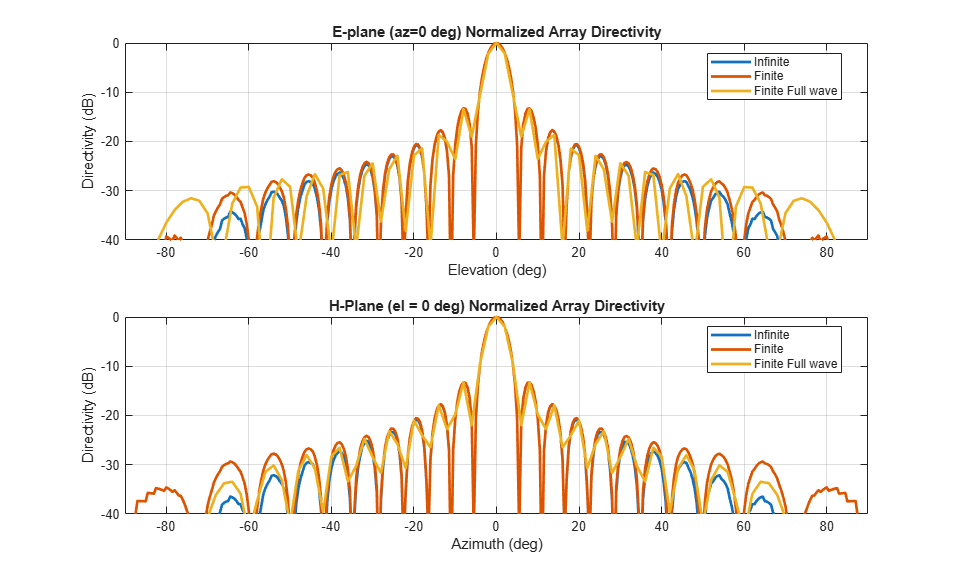The pattern plots in the two planes reveals that all three analysis approaches suggest similar behavior out to +/-40 degree from boresight. Beyond this range, it appears that using the scan element pattern for all elements in a URA underestimates the sidelobe level compared to the full wave analysis of a finite array. The one possible reason for this could be the edge effect from the finite size array.

Comparison of Element Patterns The element patterns from the infinite array analysis and the finite array analysis are compared here.

figure subplot(211) plot(elang_plot,DSEP1_Enormlz,elang_plot,DSEP2_Enormlz,'LineWidth',2) grid on axis([min(azang_plot) max(azang_plot) -40 0]); legend('Infinite','Finite','location','best') xlabel('Elevation (deg)') ylabel('Directivity (dB)') title('E-plane (az=0 deg) Normalized Element Directivity') subplot(212) plot(azang_plot,DSEP1_Hnormlz,azang_plot,DSEP2_Hnormlz,'LineWidth',2) grid on axis([min(azang_plot) max(azang_plot) -40 0]); legend('Infinite','Finite','location','best') xlabel('Azimuth (deg)') ylabel('Directivity (dB)') title('H-Plane (el = 0 deg) Normalized Element Directivity')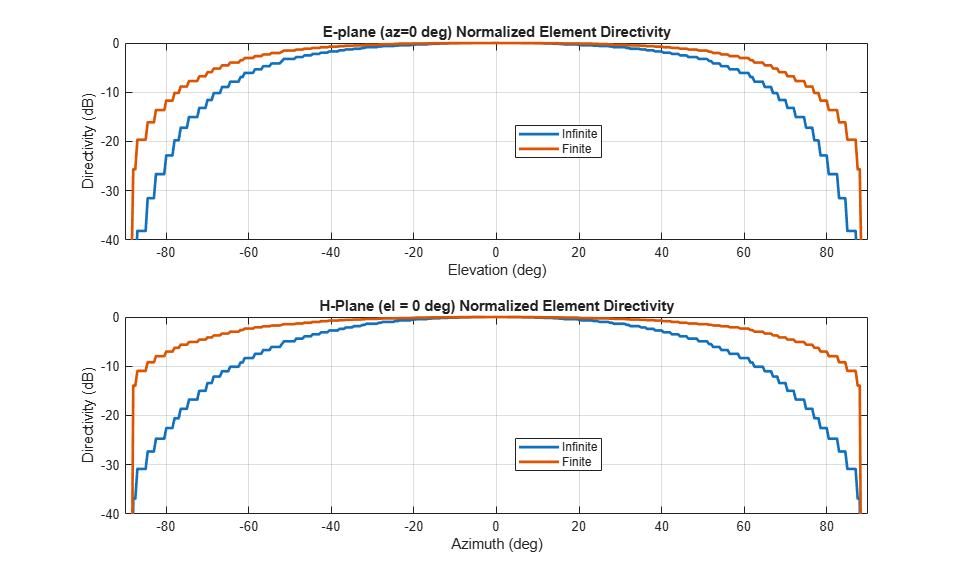### Scan Behavior with Infinite Array Scan Element Pattern

Scan the array based on the infinite array scan element pattern in the elevation plane defined by azimuth = 0 deg and plot the normalized directivity. Also, overlay the normalized scan element pattern.

helperATXScanURA(myURA1,freq,azang_plot,elang_plot,... DSEP1_Enormlz,DSEP1_Hnormlz);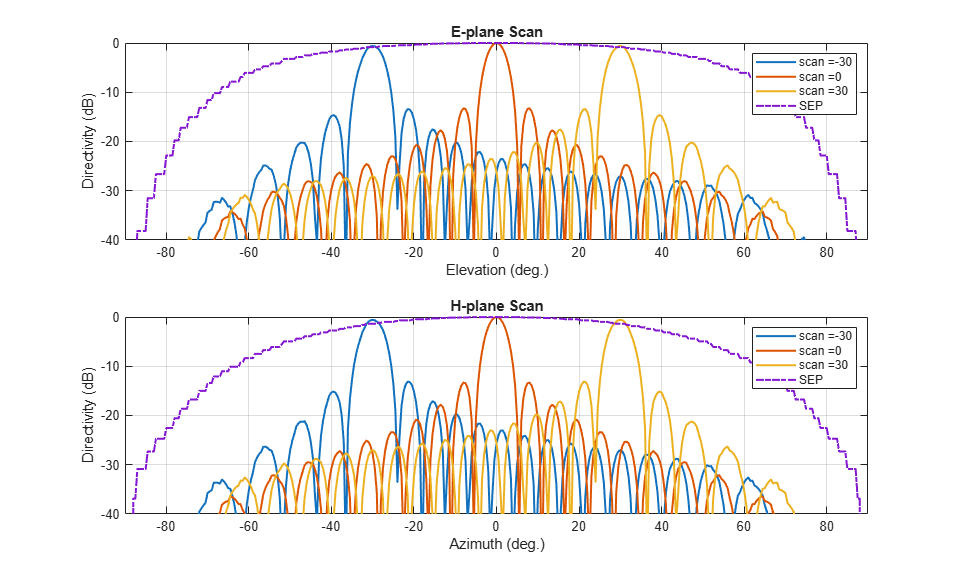Note the overall shape of the normalized array pattern approximately follows the normalized scan element pattern. This is also predicted by the pattern multiplication principle.

### Conclusion

The infinite array analysis is one of the tools deployed to analyze and design large finite arrays. The analysis assumes that all elements are identical, that edge effects can be ignored and have uniform excitation amplitude The isolated element pattern is replaced with the scan element pattern which includes the effect of mutual coupling.

### Reference

 J. Allen, "Gain and impedance variation in scanned dipole arrays," IRE Transactions on Antennas and Propagation, vol.10, no.5, pp.566-572, September 1962.

 R. C. Hansen, Phased Array Antennas, Chapter 7 and 8, John Wiley & Sons Inc.,2nd Edition, 1998.